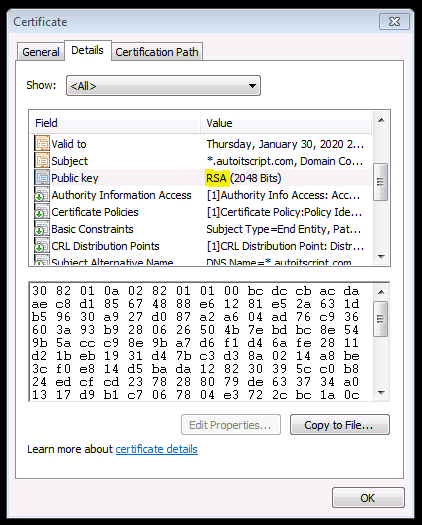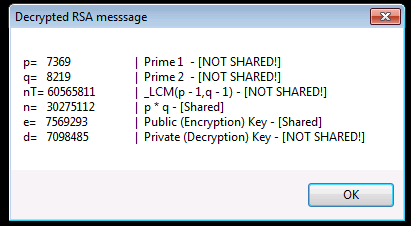# Toy RSA Encryption Example - Simple concepts for learning Public Key Encryption

## Recommended Posts

I found this article and enjoyed it so much I had play with some code since the numbers are small enough.

Standard Encryption's vs RSA Encryption (Public Key Encryption) Fundamental Differences
If you read that and couldn't immediately clarify the difference then let me blow your mind because its simple:

STANDARD ENCRYPTION'S:

ORIGINAL_DATA + Password(or KEY) = Encrypted DATA
Then to decrypt ->
Encrypted DATA + (SAME Password(or SAME KEY)) = ORIGINAL_DATA

RSA:
ORIGINAL_DATA + Password(or PUBLIC_KEY) = Encrypted DATA
Then to decrypt ->
Encrypted DATA + (DIFFERENT Password(or PRIVATE_KEY)) = ORIGINAL_DATA

Are we all caught up? Did the colors help? I think they didThat's crazy right? Don't answer. It is. And crazier its used EVERY TIME we make a secure connection to a server over the internet. But here's the craziest part to me that I recently got clarity on from the toy example and that is the simplicity of this very very very very important algorithm that has yet to be cracked (fingers crossed):

Mod(\$vData ^ \$key, \$n)

So ya. That's it. That's the magic algorithm. 3 values. Oh and \$n is also a shared known value that will be in the certificate with the public key that your browser reads when it makes a connection:That's just mind blowing to me so couldn't resist getting something going in AUT. After playing with this code, I got a much better understanding of how its not just that algorithm that makes this whole thing possible. The numbers that we pick to form the public key and n are just as important and also how important it is to be random!

Let me know if you have any problems. Enjoy!

```#include <array.au3>

_Toy_RSA_Example()

;https://thatsmaths.com/2016/08/11/a-toy-example-of-rsa-encryption/
Func _Toy_RSA_Example()

Local \$p, \$q, \$n, \$nT, \$e, \$d
Local \$aPublicKeys, \$aCrypt, \$sDecrypt, \$sMsg

;Pick two random primes (they will be between 1000-10000)
\$p = _GetRandomPrime()
\$q = _GetRandomPrime()
\$sMsg = 'p=   %i \t\t|  Prime 1  - [NOT SHARED!]\nq=   %i \t\t|  Prime 2  - [NOT SHARED!]\n'

;Calculate lowest common multiple
\$nT = _LCM(\$p - 1, \$q - 1)
\$sMsg &= 'nT= %i \t|  _LCM(p - 1,q - 1) - [NOT SHARED!]\n'

;Calculate n. This is a shared number
\$n = \$p * \$q
\$sMsg &= 'n=   %i \t|  p * q - [Shared]\n'

;Get a small random list of possible public keys to pick from. Only searching for 100ms
\$aPublicKeys = _GetPublicKeys(\$nT)
_ArrayDisplay(\$aPublicKeys, "Possible Public Keys Found")

;Pick a random public (encryption) key from array
\$e = \$aPublicKeys[Random(1, \$aPublicKeys, 1)]
\$sMsg &= 'e=   %i \t|  Public (Encryption) Key - [Shared]\n'

;Generate our private (decryption) key
\$d = _GetPrivateKey(\$e, \$nT)
\$sMsg &= 'd=   %i \t|  Private (Decryption) Key - [NOT SHARED!]\n'

;format our msg (rsa details) to encrypt
\$sMsg = StringFormat(\$sMsg, \$p, \$q, \$nT, \$n, \$e, \$d)

;encrypt message
\$aCrypt = _RSA(\$sMsg, \$e, \$n)
_ArrayDisplay(\$aCrypt, 'Encrypted RSA messsage')

;Decrypt array back
\$sDecrypt = _RSA(\$aCrypt, \$d, \$n)
MsgBox(0, 'Decrypted RSA messsage', \$sDecrypt)

EndFunc   ;==>_Toy_RSA_Example

;Function will perfrom Mod(\$v ^ \$key, \$n) on each char/element.
;Excepts Arrays or Strings. If input is array a string is returned and vice versa.
Func _RSA(\$vDat, \$key, \$n)
Local \$bIsStr = IsString(\$vDat)
If \$bIsStr Then \$vDat = StringToASCIIArray(\$vDat)
For \$i = 0 To UBound(\$vDat) - 1
\$vDat[\$i] = _Modular(\$vDat[\$i], \$key, \$n)
Next
Return \$bIsStr ? \$vDat : StringFromASCIIArray(\$vDat)
EndFunc   ;==>_RSA

;algorithm is from the book "Discrete Mathematics and Its Applications 5th Edition" by Kenneth H. Rosen.
Func _Modular(\$iBase, \$iExp, \$iMod) ;  Mod(\$v ^ \$key, \$n)
Local \$iPower = Mod(\$iBase, \$iMod)
Local \$x = 1

For \$i = 0 To (4 * 8) - 1
If BitAND(0x00000001, BitShift(\$iExp, \$i)) Then
\$x = Mod((\$x * \$iPower), \$iMod)
EndIf
\$iPower = Mod((\$iPower * \$iPower), \$iMod)
Next

Return \$x
EndFunc   ;==>_Modular

;Generate a "random" list of possible valid public keys to choose from based on \$nT
Func _GetPublicKeys(\$nT, \$iMs = 100)
Do
Local \$aKeys = , \$iTime = TimerInit()
Local \$i = (Mod(@SEC, 2) ? Int(\$nT / 2) : Int(\$nT / 4)) ; randomize where we start
Do
If _IsPrime(\$i) And _IsCoPrime(\$i, \$nT) Then
\$aKeys += 1
\$aKeys[\$aKeys] = \$i
EndIf
\$i += (Mod(@MSEC, 2) ? 1 : 100) ; randomize step size
Until (\$i >= (\$nT - 1)) Or (TimerDiff(\$iTime) > \$iMs)
ReDim \$aKeys[\$aKeys + 1]
Until \$aKeys > 5 ; Ive seen 200+ returned sometimes and 0 on others. Make sure we have at least a few choices
Return \$aKeys
EndFunc   ;==>_GetPublicKeys

;https://www.geeksforgeeks.org/multiplicative-inverse-under-modulo-m/  - _ModInverse(a,m)
Func _GetPrivateKey(\$a, \$m)
If (\$m = 1) Then Return 0 ;

Local \$t, \$q, \$y = 0, \$x = 1, \$m0 = \$m
While (\$a > 1)
\$q = Int(\$a / \$m) ;q is quotient
\$t = \$m ;

\$m = Mod(\$a, \$m) ;m is remainder now, process same as Euclid's algo
\$a = \$t ;
\$t = \$y ;

\$y = \$x - \$q * \$y ;Update y and x
\$x = \$t ;
WEnd

Return \$x < 0 ? \$x + \$m0 : \$x
EndFunc   ;==>_GetPrivateKey

;Pick the next nearest prime from a random number (or number you cho0se)
Func _GetRandomPrime(\$iStart = Default)
Local \$iPrime = (\$iStart = Default ? Random(1000, 10000, 1) : \$iStart)
Do
\$iPrime += 1
Until _IsPrime(\$iPrime)
Return \$iPrime
EndFunc   ;==>_GetRandomPrime

#Region Math Functions

Func _IsPrime(\$n)
For \$i = 2 To (Int(\$n ^ 0.5) + 1)
If Mod(\$n, \$i) = 0 Then Return False
Next
Return True
EndFunc   ;==>_IsPrime

Func _IsCoPrime(\$a, \$b)
Return _GCD(\$a, \$b) = 1
EndFunc   ;==>_IsCoPrime

Func _GCD(\$iX, \$iY)
Local \$iM
While 1
\$iM = Mod(\$iX, \$iY)
If \$iM = 0 Then Return \$iY
\$iX = \$iY
\$iY = \$iM
WEnd
EndFunc   ;==>_GCD

Func _LCM(\$iX, \$iY)
Return (\$iX * \$iY) / _GCD(\$iX, \$iY)
EndFunc   ;==>_LCM

#EndRegion Math Functions```

You should get a message box displaying the decrypted message with details of the values used:Edited by Beege
##### Share on other sites

For those who are interestd in go a little bit deeper, I think Khan academy has done a topic (with videos) about it, that is where I learned about how RSA works and how it was independently rediscovered.

A little bit of math logic and prime numbers you can have a good way to encrypt data without a single shared key. Asymmetrical encryption they call it I think...

I found the topic: Journey into cryptography, it covers RSA in the modern cryptography section: https://www.khanacademy.org/computing/computer-science/cryptography#modern-crypt

EasyCodeIt - A cross-platform AutoIt implementation - Fund the development! (GitHub will double your donations for a limited time)

##### Share on other sites

5 minutes ago, TheDcoder said:

I found the topic: Journey into cryptography, it covers RSA in the modern cryptography section: https://www.khanacademy.org/computing/computer-science/cryptography#modern-crypt

Nice thank you! I love a lot of topics on that page##### Share on other sites

For those who would want to experiment with larger numbers (not too large though) you can use the _BigNum_PowerMod function I've posted somewhere.

Here it is, with a BigNum prime test (good enough for experimentation but unsuitable for military grade application):

Function names should be instead _BigNum_PowerMod (only one leading _) and _BigNum_IsComposite for consistancy.

_BigNum_GCD() and _BigNum_LCM() left as a trivial exercise.

Edited by jchd

This wonderful site allows debugging and testing regular expressions (many flavors available). An absolute must have in your bookmarks.
RegExp tutorial: enough to get started
PCRE v8.33 regexp documentation latest available release and currently implemented in AutoIt beta.

SQLitespeed is another feature-rich premier SQLite manager (includes import/export). Well worth a try.
SQLite Expert (freeware Personal Edition or payware Pro version) is a very useful SQLite database manager.
An excellent eBook covering almost every aspect of SQLite3: a must-read for anyone doing serious work.
SQL tutorial (covers "generic" SQL, but most of it applies to SQLite as well)
A work-in-progress SQLite3 tutorial. Don't miss other LxyzTHW pages!
SQLite official website with full documentation (may be newer than the SQLite library that comes standard with AutoIt)

## Create an account

Register a new account

• ### Similar Content

• #### Simple encryption based on the Enigma Machine

By willichan,

• 6 replies
• 583 views
• #### CryptoNG UDF - Cryptography API: Next Generation

By TheXman,

• 9,997 views
• #### CodeCrypter - Encrypt your Script 1 2 3 4 22

By RTFC,

• 425 replies
• 121,334 views
• #### CodeScannerCrypterBundle

By RTFC,

• 6,024 views
• #### MCF - MetaCode File UDF

By RTFC,

• 18 replies
• 16,849 views
×

• Wiki

• Back

• #### Beta

• Git
• FAQ
×
• Create New...